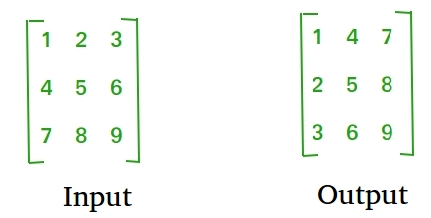GeeksforGeeks App
Open AppBrowser
Continue

# Python Program to find transpose of a matrix

Transpose of a matrix is obtained by changing rows to columns and columns to rows. In other words, transpose of A[][] is obtained by changing A[i][j] to A[j][i].For Square Matrix: The below program finds transpose of A[][] and stores the result in B[][], we can change N for different dimension.

## Python3

 `# Python3 Program to find``# transpose of a matrix` `N ``=` `4` `# This function stores``# transpose of A[][] in B[][]` `def` `transpose(A,B):` ` ``for` `i ``in` `range``(N):``  ``for` `j ``in` `range``(N):``   ``B[i][j] ``=` `A[j][i]` `# driver code``A ``=` `[ [``1``, ``1``, ``1``, ``1``],`` ``[``2``, ``2``, ``2``, ``2``],`` ``[``3``, ``3``, ``3``, ``3``],`` ``[``4``, ``4``, ``4``, ``4``]]`  `B ``=` `A[:][:] ``# To store result` `transpose(A, B)` `print``(``"Result matrix is"``)``for` `i ``in` `range``(N):`` ``for` `j ``in` `range``(N):``  ``print``(B[i][j], ``" "``, end``=``'')`` ``print``()`` ` `# This code is contributed by Anant Agarwal.`

Output:

```Result matrix is
1  2  3  4
2  2  3  4
3  3  3  4
4  4  4  4```

Time Complexity: O(n2)
Auxiliary Space: O(n2)

For Rectangular Matrix: The below program finds transpose of A[][] and stores the result in B[][].

## Python3

 `# Python3 Program to find``# transpose of a matrix` `M ``=` `3``N ``=` `4` `# This function stores``# transpose of A[][] in B[][]` `def` `transpose(A, B):` ` ``for` `i ``in` `range``(N):``  ``for` `j ``in` `range``(M):``   ``B[i][j] ``=` `A[j][i]` `# driver code``A ``=` `[ [``1``, ``1``, ``1``, ``1``],`` ``[``2``, ``2``, ``2``, ``2``],`` ``[``3``, ``3``, ``3``, ``3``]]`  `# To store result``B ``=` `[[``0` `for` `x ``in` `range``(M)] ``for` `y ``in` `range``(N)]` `transpose(A, B)` `print``(``"Result matrix is"``)``for` `i ``in` `range``(N):`` ``for` `j ``in` `range``(M):``  ``print``(B[i][j], ``" "``, end``=``'')`` ``print``() `

Output:

```Result matrix is
1  2  3
1  2  3
1  2  3
1  2  3```

Time Complexity: O(n*m)
Auxiliary Space: O(n*m)

In-Place for Square Matrix:

## Python3

 `# Python3 Program to find``# transpose of a matrix` `N ``=` `4` `# Finds transpose of A[][] in-place``def` `transpose(A):` ` ``for` `i ``in` `range``(N):``  ``for` `j ``in` `range``(i``+``1``, N):``   ``A[i][j], A[j][i] ``=` `A[j][i], A[i][j]` `# driver code``A ``=` `[ [``1``, ``1``, ``1``, ``1``],`` ``[``2``, ``2``, ``2``, ``2``],`` ``[``3``, ``3``, ``3``, ``3``],`` ``[``4``, ``4``, ``4``, ``4``]]` `transpose(A)` `print``(``"Modified matrix is"``)``for` `i ``in` `range``(N):`` ``for` `j ``in` `range``(N):``  ``print``(A[i][j], ``" "``, end``=``'')`` ``print``()`` ` `# This code is contributed by Anant Agarwal.`

Output:

```Modified matrix is
1  2  3  4
1  2  3  4
1  2  3  4
1  2  3  4```

Time Complexity: O(n2)
Auxiliary Space: O(1)

Please refer complete article on Program to find transpose of a matrix for more details!

#### Approach#4: Using zip()

In this approach, zip(*matrix) is used to “unzip” the rows of the matrix and group the corresponding elements together. The * operator is used to pass the rows of the matrix as separate arguments to zip(). The resulting tuples are then converted back into lists using a list comprehension.

#### Algorithm

1. Define the matrix to be transposed.
2. Use the zip() function to group the corresponding elements of each row together and create tuples from them.
3. Convert each tuple back to a list using a list comprehension.
4. Store the resulting list of lists as the transposed matrix.
5. Print both the original and transposed matrices.

## Python3

 `matrix ``=` `[[``1``, ``1``, ``1``, ``1``],``          ``[``2``, ``2``, ``2``, ``2``],``          ``[``3``, ``3``, ``3``, ``3``],``          ``[``4``, ``4``, ``4``, ``4``]]` `transpose ``=` `[``list``(row) ``for` `row ``in` `zip``(``*``matrix)]` `print``(``"Original Matrix:"``)``for` `row ``in` `matrix:``    ``print``(row)` `print``(``"Transposed Matrix:"``)``for` `row ``in` `transpose:``    ``print``(row)`

Output

```Original Matrix:
[1, 1, 1, 1]
[2, 2, 2, 2]
[3, 3, 3, 3]
[4, 4, 4, 4]
Transposed Matrix:
[1, 2, 3, 4]
[1, 2, 3, 4]
[1, 2, 3, 4]
[1, 2, 3, 4]```

Time Complexity:
The time complexity of this program is O(n^2) where n is the number of rows or columns in the matrix. This is because we need to access each element of the matrix exactly once to create the transposed matrix.

Space Complexity:
The space complexity of this program is also O(n^2). This is because we need to store the original matrix and the transposed matrix in memory, which both have n^2 elements. Additionally, we create temporary tuples during the transposition process, but these are discarded after they are converted back to lists.

METHOD 5:Using list comprehension

APPROACH:

This program demonstrates how to find the transpose of a given matrix using a list comprehension.

ALGORITHM:

1.Initialize a 2D list A with the given matrix values.
2.Create a new 2D list result using a nested list comprehension.
3.In the inner list comprehension, iterate through the rows of A and extract the ith element from each row.
4.Append the extracted elements as a row to the result list.
5.Print the result matrix by iterating through each row and joining the elements with a space.

## Python3

 `A ``=` `[[``1``, ``1``, ``1``, ``1``],``     ``[``2``, ``2``, ``2``, ``2``],``     ``[``3``, ``3``, ``3``, ``3``],``     ``[``4``, ``4``, ``4``, ``4``]]` `result ``=` `[[row[i] ``for` `row ``in` `A] ``for` `i ``in` `range``(``len``(A[``0``]))]` `# Print the result``for` `row ``in` `result:``    ``print``(``' '``.join([``str``(elem) ``for` `elem ``in` `row]))`

Output

```1 2 3 4
1 2 3 4
1 2 3 4
1 2 3 4```

Time complexity: O(n^2) – as the program iterates through each element of the matrix A and creates a new matrix of the same size.

Auxiliary Space: O(n^2) – as the program creates a new matrix of the same size as A to store the transpose.

My Personal Notes arrow_drop_up